Control Index

Nyquist Stability graphs

Polar graphs

There are a number of polar graph options for studying control systems including the nyquist, inverse polar plot and the nichols plot.  The nyquist open loop polar plot indicates the degree of stability, and the adjustments required and provides stability information for systems containing time delays.  Polar plots are not used exclusively because,without powerful computing facilities, they can be difficult to generate at a detailed level and they do not directly yield frequency values.

The Nyquist plot is obtained by simply plotting a locus of imaginary(G(j ω)) versus Real(G(j ω)) at the full range of frequencies from ( - ¥ to + ¥ ) It is very easy to produce nyquist plots by hand or by using proprietary software packages such as Matlab.   Links below show how bode and nyquist plots can be produced using Excel and using Mathcad.  The plots below have been produced in minutes using Mathcad..

The nyquist plot fundamentals are shown below....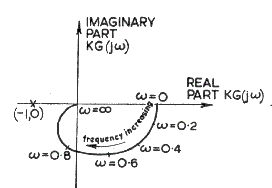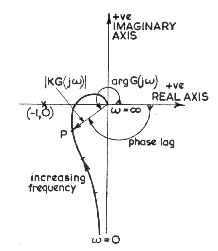Velocity Feedback control

Basic Rules for constructing Nyquist plots

In control systems a transfer function to be assessed is often of the form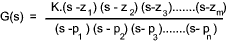This transfer function is modified for frequency response analysis by replacing the s with jω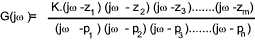Assuming the function is proper and n > m..he Nyquist plot will have the following characteristics. Crude plots to be may be produced relatively easily using these characteristics.

 Asymptotic behavior.. For n - m > 1, the Nyquist plot approaches the origin at an asymptotic angle of -(n-m) p/2... Assuming G(s) = K(s)/s k.  For k poles at zero, the Nyquist plot comes in from infinity at an angle of -(n-m) p/2 In a system with no OL zeros, the plot of G(jω) will decrease monotonically as ω rises above the level of the largest imaginary part of the poles; This will also be true for large enough ω even in the presence of zeros.(Ref plot 1 below). The plot will cross the imaginary axis when Real G(jω) =0 and will cross the real axis when Imaginary G(jω) = 0,   ( for crossing of the negative real axis use Arg- G(jω)= p )

Relative stability assessments Using the Nyquist Plot

As identified in the page on frequency response Frequency response The nyquist plots are based on using open loop performance to test for closed loop stability.  The system will be unstable if the locus has unity value at a phase crossover of 180 o ( p ).

Two relative stability indicators "Gain Margin" and "Phase Margin" may be determined from the suitable Nyquist Plots.   The degree of gain margin is indicated as the amount the gain is less than unity when the plot crosses the 180 o axis (Phase crossover). The phase margin is the angle the phase is less than 180 o when the gain is unity.  The values are generally identified by use of Bode plots

The phase margin is clearly illustrated below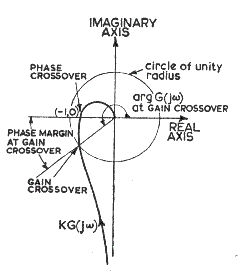Nyquist Stability Criterion.

In the nyquist plots below the area covered to the right of the locus(shaded) is the Right Hand Plane (RHP)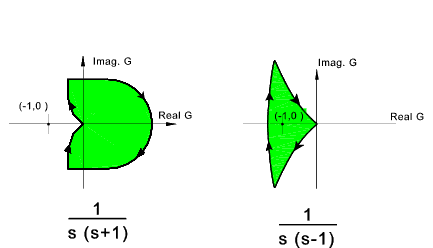A closed loop control system is absolutely stable if the roots of the characteristic equation have negative real parts.  This means the poles of the closed loop transfer function, or the zeros of the denominatior ( 1 + GH(s)) of the closed loop tranfer function, must lie in the (LHP).   The nyquist stability criterion establishes the number of zeros of (1 + GH(s) in the RHP directly from the Nyquist stability plot of GH(s) as indicated below.

The closed loop control system whose open loop transfer function is GH(s) is stable only if..

N = -Po ≤ 0

where
1) P o = the number of G(s) poles in the RHP ³ 0
2) N = total number of CW encirclements of the (-1,0) in the G(s) plane.

If N > 0 the number of zeros (Z o) in the RHP is determined by Z o = N + P o

If N 0 the (-1,0) point is not enclosed by the nyquist plot.
If N and P 0 then the system is absolutely stable only if N = 0.   That is if and only if the (-1,0) point does not lie in the shaded region..

Considering the LH plot above of 1/s(s+1). The (-1,0) point is not in the RHP therefore N<= 0. The poles are at s =0, and s=-1, both outside of the RHP and therefore P o = 0.
Thus N = -P o = 0 and the system is therefore stable.

Considering the RH plot above of 1/s(s-1).   The (-1,0) point is enclosed in the RHP and therefore N > 0 (N= 1).  The poles of GH are at s= 0 and s = +1 .   S= +1 is in the RHP and therefore P o = 1.
N ¹ - P o Indicating that this system is unstable..

There are Z o = N + P o zeros of 1+GH in the RHP.

Nyquist Plots A number of typical nyquist plots are shown below to illustrate the various shapes.

Plot 1..... 1 /(s + 2)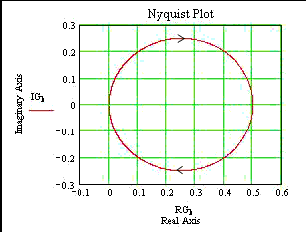1 /(s + 2)

Note that G(i0) = 0.5 and as ω increases to ¥ the plot approaches zero along the negative locus.
G(jω) moves from 0 to 0.5 as ω - ¥ to 0
G(j ¥) = 0
The asymptotic angle approaching 0 is = -90 o and

Plot 2.....1 /(s 2 + 2s + 2)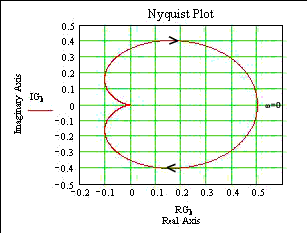1 /(s 2 + 2s + 2)

The zero-frequency behaviour is:G(j0) =0.5
G(j ¥) = 0
The asymptotic angle is = -180 o

Plot 3.....s(s+1) /(s 3 + 5.s 2 +3.s + 4 )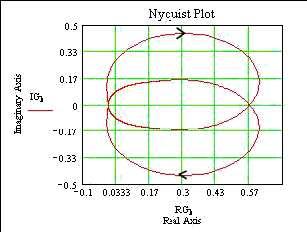s(s+1) /(s 3 + 5.s 2 +3.s +4 )

Plot 4.....(s+1) /[(s+2)(s+3)]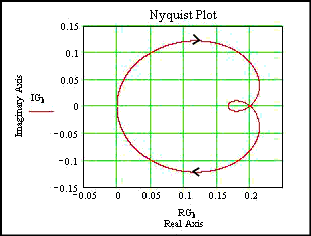((s+1) /[(s+2)(s+3)]

Plot 5.....1 /s(s-1).. an unstable regime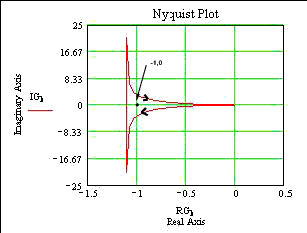(1 /s (s-1)

 Sites & Links For Control Information Michigan Tech Uni. Paper..Tutorial On using Mathcad to create Bode & Nyquist Plots Michigan Tech Uni. Paper..Tutorial On using Excel to create Bode & Nyquist Plots Review of ~Frequency response analysis ..Reviews the different method including bode , nyquist etc Control system design based on frequency response analysis ..Extremely clear document Nquist Plots..Short set of notes with grphed examples econtrolhtml

Control Index